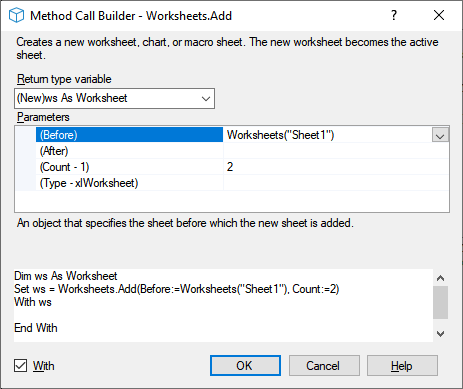﻿ Create new worksheet using VBA

# Add new worksheet with nameThe code below shows how you can create a new Worksheet and give it a name. Note that with Add the new sheet always becomes the active sheet.

``````Dim wb As Workbook: Set wb =
Dim strName As String: strName =
Dim ws As Worksheet
With ws
.Name = strName
End With``````

The below sections discuss the arguments you can use to control how worksheets are added with specified position: Before and After, Count and what Type possibly using a template Template. At the end there is a macro you can use to Insert sheet with name specified by the user.

### Before or After arguments

An object that specifies the sheet Before or After which the new sheet is added. If Before and After are both omitted, the new sheet is inserted before the active sheet.

As an example, below code adds a new worksheet After the active sheet in the active workbook

``````Dim wb As Workbook
Set wb = ActiveWorkbook
Dim ws As Worksheet

The following code puts the new sheet at the beginning:

``Set ws = wb.Worksheets.Add(Before:=wb.Worksheets(1), Type:=xlWorksheet)``

The following code puts the new sheet at the end:

``Set ws = wb.Worksheets.Add(After:=wb.Worksheets(wb.Worksheets.Count), Type:=xlWorksheet)``

### Count

The number of sheets to be added. The default value is one.

### Type

Specifies the sheet type. Can be one of the following XlSheetType constants: xlWorksheet or xlChart - or template file path. The default value is xlWorksheet.

#### Use Template

You can also insert sheets based on an existing template, see below.

``````Dim wb As Workbook: Set wb = ActiveWorkbook
Dim ws As Worksheet
Dim strTemplate As String: strTemplate = "C:\temp\tpt.xlsx"

## Insert sheet with name specified by the user

If you often have to insert a worksheet and give it a name the macro below can be handy. The code is build up as follows:

1. Uses `InputBox` to ask for the name of the worksheet to be inserted
• The `Title` argument gives the pupose of the current action;
• The `Prompt` argument specifies what input is required. Some details are added concerning not allowed characters
• You could use `Default` argument to automatically fix errors in previously not ok sheet name, or pick up a proposed name somewhere else.
2. Validates the string returned by the  `InputBox` :
1. If empty string the sheet insertion is aborted.
2. If there already is a sheet with this name
3. If the string does not comply with excel sheet naming rules
a `MsgBox` informs the user about any problems and lets the user either Abort or Retry.
3. Inserts a worksheet and gives it the name specified in the inputbox.
``````Sub AddNewWorksheet()
Const cstrTitle As String = "Add new worksheet"
Const cstrPrompt As String = "Give the name for the new worksheet." & vbCrLf & "Not allowed are the characters: : \ / ? * [ and ]"
Dim strInput As String
Dim strDefault As String: strDefault = "" 'setting initial value for inputbox can be useful
Dim strInputErrorMessage As String
Dim booValidatedOk As Boolean: booValidatedOk = False
On Error GoTo HandleError

Do
strInput = InputBox(Prompt:=cstrPrompt, Title:=cstrTitle, Default:=strDefault)
If Len(strInput) = 0 Then GoTo HandleExit
GoSub ValidateInput
If Not booValidatedOk Then
If vbCancel = MsgBox(strInputErrorMessage & "Retry?", vbExclamation + vbOKCancel) Then GoTo HandleExit
End If
Loop While Not booValidatedOk

Dim wb As Workbook: Set wb = ActiveWorkbook
Dim shts As Sheets: Set shts = wb.Sheets
Dim obj As Object
Set obj = shts.Add(Before:=ActiveSheet, Count:=1, Type:=XlSheetType.xlWorksheet)
obj.Name = strInput

HandleExit:
Exit Sub
HandleError:
MsgBox Err.Description
Resume HandleExit

ValidateInput:
If SheetExists(strSheetName:=strInput) Then
strInputErrorMessage = "Sheet already exists. "
ElseIf Not IsValidSheetName(strSheetName:=strInput) Then
strInputErrorMessage = "Sheetname not allowed. "
Else
booValidatedOk = True
End If
Return
End Sub``````